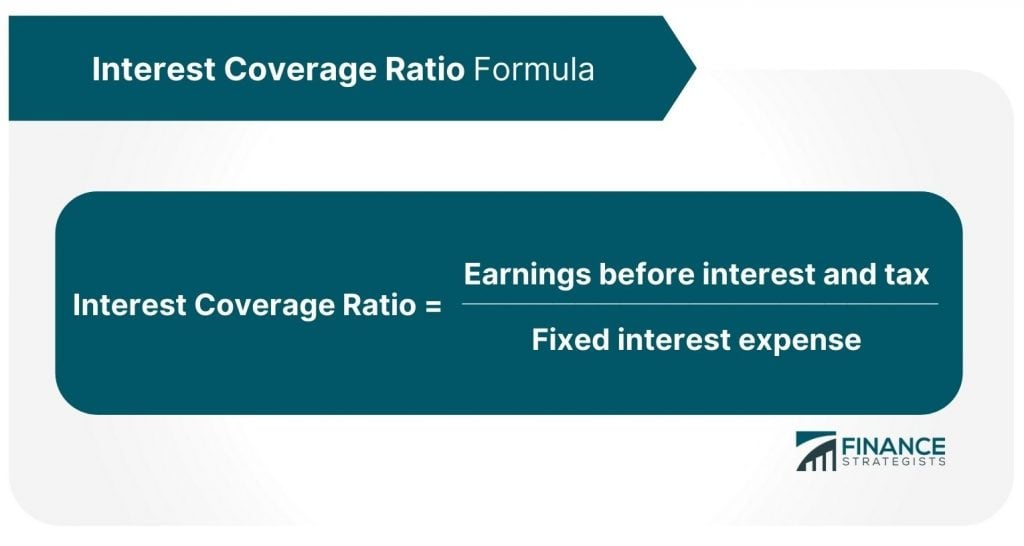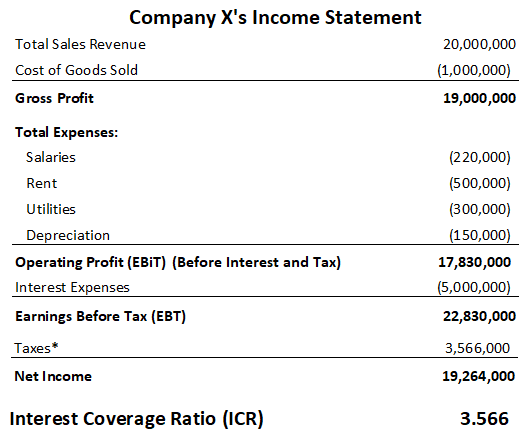# Interest Coverage Ratio (ICR)### Written byTrue Tamplin, BSc, CEPF®

Updated on March 29, 2022

## Interest Coverage Ratio (ICR): Definition

The interest coverage ratio (ICR) indicates how well a company can service its long-term loans. The ICR is calculated by dividing net profit (before deducting the interest) by the total interest expenses.

The ICR is expressed in times. Times interest earned or ICR is a measure of a company’s ability to honor its debt payments.

The ICR is also known as the debt service ratio or debt service coverage ratio. It may be calculated as either EBIT or EBITDA divided by the total interest expense of the company.

## Interest Coverage Ratio: Explanation

The interest coverage ratio (ICR) is an important and much-studied ratio. This is especially true when borrowing is high relative to shareholder funds.

This situation, known as being highly geared, is explained here. It is also particularly significant when the interest charge is high relative to profits.

Obviously, a company that cannot pay its interest charge has severe problems and might not be able to carry on, at least not without a fresh injection of funds.

The ICR is profit before interest and tax divided by the interest charge. The higher the ratio, the more easily the business will manage to pay the interest charge.

## Interpretation of Interest Coverage Ratio

If a company’s interest coverage ratio (ICR) is high, it shows that interest payments are not a major part of the company’s total expenses. The company, therefore, is likely to be able to service its interest payments comfortably.

However, if the ICR is low, it means that even a small drop in the company’s level of operations can make the payment of interest difficult for the company. For this reason, lenders pay close attention to the ICR.

A highly geared company (i.e., a company with a high level of borrowings), will generally have a low ICR. Conversely, a low-geared company will generally have a high ratio.

## Formula to Calculate Interest Coverage Ratio

The formula for the interest coverage ratio (ICR) is written as follows:In this formula, the variables are:

• Earnings before interest and tax: The company's operating profit
• Fixed interest expenses: Interest payable on borrowings (e.g., bonds, loans, etc.)

## Example 1

The following information was extracted from the income statement of John Trading Company:

• Interest expenses: \$25,000
• Earnings before interest and tax: \$300,000

Required: Calculate John Trading Company’s interest coverage ratio (ICR).

### Solution

Interest coverage ratio = Earnings before interest and tax / Fixed interest expenses = \$300,000 / \$25,000 = 12 times

The earnings are 12 times greater than the interest expenses at John Trading Company. This shows that the company can comfortably cover the payments for interest expenses on its borrowings.

## Example 2

• Revenue = \$20,000,000
• Cost of goods sold = \$10,000,000
• Operating expenses:
• Salaries = \$220,000
• Rent = \$500,000
• Utilities = \$300,000
• Depreciation = \$150,000
• Interest expenses = \$3,000,000

Required: Prepare the company’s income statement and calculate the interest coverage ratio (ICR).

### Solution

The income statement of Company X is shown below.* The company’s annual income tax expense is 20%.

## Interest Coverage Ratio Calculator

[show_file file=”webcalculators/ratios/times-interest-earned-ratio.txt”]

## Interest Coverage Ratio (ICR) FAQs